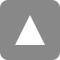﻿ The 55th Statistical Machine Learning Seminar (online) | 統計数理研究所

# The 55th Statistical Machine Learning Seminar (online)

【Date & Time】
Mar. 17, 2023 (Fri) 13:00 - 14:30

【Place】
Zoom(Online)
https://forms.gle/ZXLDNYeM5G9A2asN7
【Speaker】
Piotr Graczyk (Université d'Angers)
【Title】
Pattern Recovery by Penalized Estimators with Polyhedral Penalty
【Abstract】
There are many Penalized Least Squared Estimators (LASSO, Fused, Clustered and Generalized LASSO, SLOPE...) intensely used in 21st century Statistical Machine Learning for the recovery of information on the unknown regression parameter $\beta$ in the regression problem $Y=X\beta +\varepsilon$.

For most of them, the penalty term $\lambda {\rm pen}(b)$ in the optimization problem
$$\hat\beta:= {\rm Argmin}_{b \in R^p} \frac{1}{2} \|y - Xb\|_2^2 + \lambda {\rm pen}(b)$$
is a real-valued polyhedral norm or gauge.

For LASSO, the penalty is the $l^1$ norm. It is well known that the LASSO estimator recovers well the sign of the parameter vector $\beta$, e.g. $sign(0, 5,-6, -5, 0,6)=(0, 1,-1, -1, 0,1)$.

Any Penalized Least Squared Estimator tends to recover a pattern of the parameter vector $\beta$.

For SLOPE, the penalty is a sorted $l^1$ norm wih a tuning vector $\Lambda\in R^p$. It was observed that SLOPE recovers the signed hierarchy $h(\beta)= rank(|\beta|)\times sign(\beta)$, e.g. $h(0, 5,-6, -5, 0,6)=(0, 1,-2, -1, 0,2)$. Thus SLOPE may eliminate, like LASSO, irrelevant predictors and may identify groups of predictors having the same influence on the vector of responses.

We identify the pattern of a Penalized Estimator as a common subdifferential $\partial {\rm pen} (b)$ of the penalty.

This novel definition of a pattern opens a way of mathematical investigations on the pattern recovery by a Penalized Estimator.

We give a practical necessary and sufficient condition for SLOPE pattern recovery of an unknown parameter $\beta$.